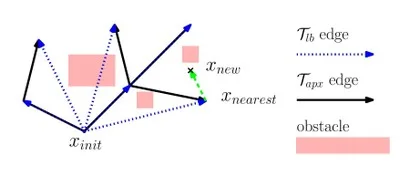# Asymptotically near-optimal RRT for fast, high-quality, motion planning

## AbstracLBT-RRT roadmaps: Two trees are simultaneously maintained by our algorithm. A lower bound (T_lb) tree and an approximation tree. The cost for reaching a node using the (possibly non collision-free) edges of the T_lb will serve as a lower bound for the optimal cost. The second tree, T_apx maintains collision-free nodes that approximate the optimal cost.

We present Lower Bound Tree-RRT (LBT-RRT), a novel, single-query sampling-based algorithm that is asymptotically near-optimal.
Namely, the solution extracted from LBT-RRT converges to a solution that is within an approximation factor of $1+\varepsilon$ of the optimal solution. Our algorithm allows for a continuous interpolation between the fast RRT algorithm and the asymptotically optimal RRT* and RRG algorithms. When the approximation factor is 1 (i.e., no approximation is allowed), LBT-RRT behaves like the RRT* algorithm. When the approximation factor is unbounded, LBT-RRT behaves like the RRT algorithm. In between, LBT-RRT is shown to produce paths that have higher quality than RRT would produce and run faster than RRT* would run.

This is done by maintaining a tree which is a sub-graph of the RRG roadmap and a second, auxiliary tree, which we call the lower-bound tree. The combination of the two trees, which is faster to maintain than the tree maintained by RRT*, efficiently guarantee asymptotic near-optimality.

We demonstrate the performance of the algorithm for scenarios ranging from 3 to 12 degrees of freedom and show that even for small approximation factors, the algorithm produces high-quality solutions (comparable to RRT*)  with little runtime overhead when compared to RRT.

Experimental results*:
Benchmark scenarios. The start and goal configuration are depicted in green and red, respectively

Success rate for algorithms on different scenarios.

Path lengths for algorithms on different scenarios

* Implemented using OMPL, the cubicles and the maze scenarios are provided with the OMPLdistribution.

• Oren Salzman,  and Dan Halperin.
Asymptotically near-optimal RRT for fast, high-quality, motion planning,
In International Conference on Robotics and Automation (ICRA), 2014

Oren Salzman
Dan Halperin